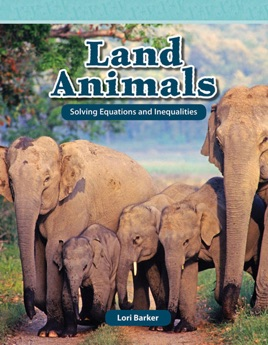• \$11.99

## Publisher Description

Practice solving equations and inequalities while learning about land animals from all over the world! Readers will learn interesting facts about earth's land animals, discovering how zoologists use variables to solve equations and inequalities and answer questions about these animals. Fun examples allow children to become familiar with functional equations, equivalent equations, inequalities, and algebraic expression. Featuring eye-catching images, exciting practice problems, easy-to-read text, STEM themes, clear mathematical charts and diagrams, and an accessible glossary and index, this title will engage students and simplify advanced mathematical concepts!